Our World Statistics Day conversations have been a great reminder of how much statistics can inform our lives. Do you have an example of how statistics has made a difference in your life? Share your story with the Community!
Choose Language Hide Translation Bar
Benefits of experiment design using random blocks

“The most commonly used class of experimental design in many industrial laboratories is the two-level factorial.” – Greenfield (1976). This bold statement was true in 1976, and I would not be surprised if were still true today. Certainly, two-level factorial designs are a standard feature in a first course in experiment design. After learning the full factorial design, a student then learns ways to avoid running every possible combination of factor settings through the use of fractional factorial designs. The next subject is often how to run fractional factorial designs in groups of runs (or blocks).

The Screening Designer in JMP provides orthogonally blocked designs for cases where the number of runs in each block is a power of 2 (i.e., 2, 4, 8, 16, etc.). Both the screening designer and most texts that introduce blocked screening designs treat the blocks as fixed rather than random.

What is the difference between fixed and random blocks?

An important difference between fixed and random blocks is how they enter the mathematical model. A fixed block is treated like a categorical factor with as many levels as there are blocks. An experiment with a fixed blocking factor having 8 blocks requires the fitting of 7 parameters.

By contrast, a random blocking factor requires the fitting of only one extra parameter, the block variance. So, the obvious benefit of random blocks is the ability to fit more factor effects with a given number of runs.

Can you give an example?

To be specific, consider an experimental scenario involving four factors where it is possible to perform two runs every day. The engineering team feels that the runs taking place on any given day are more like each other than they are like runs performed on other days. So, they want to remove any day-to-day effect by blocking the design. The team could use a standard design available through the JMP screening design tool. Figure 1 shows the designs choices for four factors. The last choice, a full factorial in 8 blocks of size 2 matches our scenario.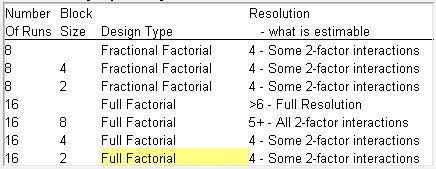Note, however that the design is Resolution IV. This means that all the two-factor interactions are not independently estimable. This is understandable when you think of how many effects there are to estimate. As I mentioned before, there are 7 block parameters. The main effects model requires another 5 parameters – one for the constant term and 4 more for each linear factor effect. There are 6 two-factor interactions, so there 18 parameters in a model containing all these effects. You cannot fit 18 unknowns with only 16 runs. It turns out that the standard design confounds the two-factor interactions with the fixed block effects.

How is using random blocks going to help?

It seems like the engineering team has an insurmountable problem if they want to estimate all the two-factor interactions and still group their experiments into blocks of two runs per day. Random blocks to the rescue!

There are only 11 parameters involving factor effects in the fixed block model. The engineering team can treat the day-to-day variation on the 8 days of the experiment as a random sample from a population of days. The model for this requires an estimate for the run-to-run variance within days and also an estimate for the day-to-day process variance. Thus, our random blocks model has 13 unknown parameters, which is conveniently less than the number of runs budgeted.

This approach sounds too good to be true.

Usually when things sound too good to be true, they are. But try following the steps below on your own to convince yourself that this is really workable.

The Custom Designer in JMP has a facility for creating optimal random block designs. Figure 2 shows the set-up for our scenario.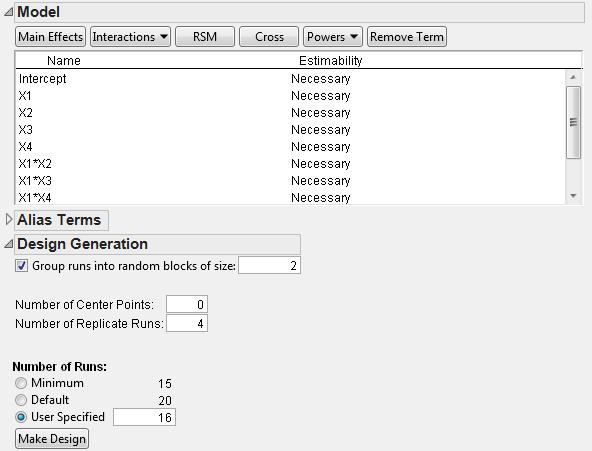You can see that the model includes all the main effects and two-factor interactions. I checked the box under the Design Generation outline node to group my runs in to random blocks of size 2. Note that I also asked for 4 replicated runs. This is very useful in this case because it allows for better estimates of the pure error variance and the block variance. Table 1 shows the resulting design.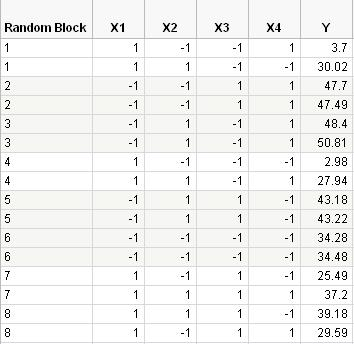I generated the values for the responses (Y column) using the Simulate Responses choice off the red triangle menu of the Custom Designer. When you make the table, you see a window containing the values for the coefficients.

Here is the true prediction equation:

Y = 34 - 9 X1 + 6 X2 + 5 X3 + 3 X1 X2 + 3 X1 X3 - 4 X2 X3                        1)

I added independent random normal errors for with a standard deviation of 2 to each block and separately independent random normal error with a standard deviation of 1 to each run.

Note that all the model terms involving the factor X4 are missing. That is, X4 is not active.

Table 2 shows the resulting Parameter Estimates.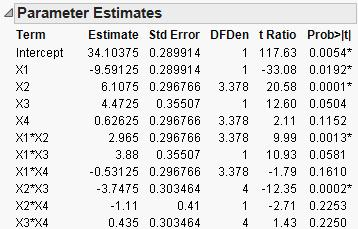The coefficients of all the terms containing X4 are not significant. If we remove them from the model, we get the reduced model shown in Table 3.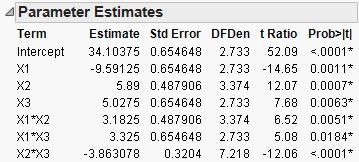Compare the fitted coefficients with their true values in equation 1.

The bottom line…

Optimal random blocking designs are new and potentially very useful. This is especially so when you have blocks with only 2 or 3 runs. Using fixed blocks means that you lose one run per block to estimate the fixed block effect. This loss of one run per block is not necessary if you use random blocks. That means that you can fit a larger model with a smaller number of runs saving time and money.

Article Labels

There are no labels assigned to this post.

Article Tags
1 Comment
Visitor

Stu Hunter wrote: